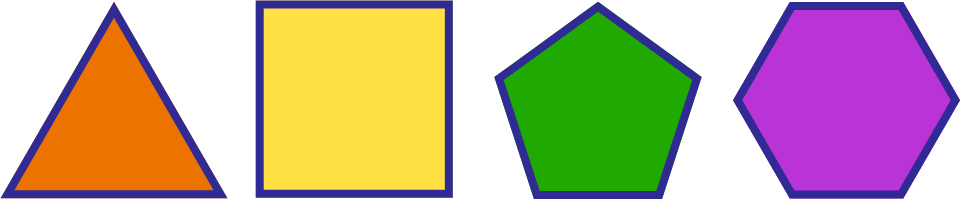# Polygonal Angles 1 – Angle Sum

Geometry Level 1Regular $n$-gon Internal Angle Sum 3 180 4 360 5 540 6 720

Given the pattern seen in this table, which of the following accurately represents the internal angle sum in terms of $n$?

×